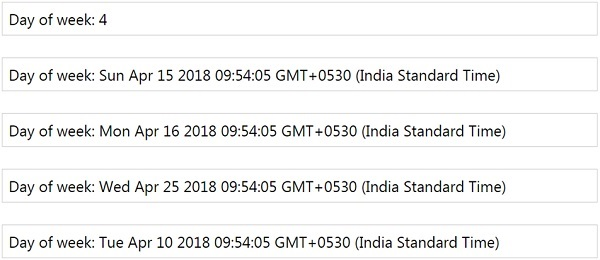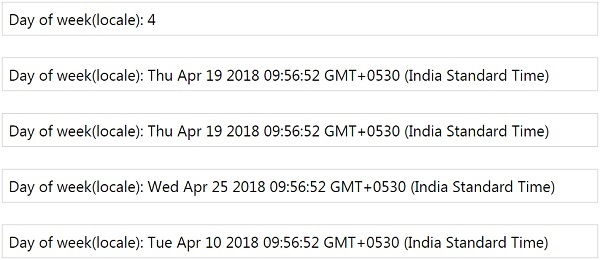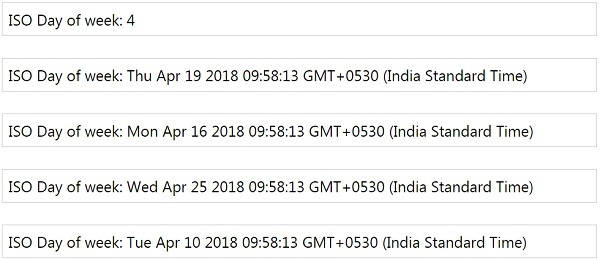# MomentJS - Day of week

This method will get/set the day of the week. It takes input from 0-6, where 0 is for Sunday and 6 as Saturday. If the value is greater than the range, it will fall in the next week. You can set the day of week using number or string.

### Syntax

```moment().day(Number|String);
moment().day();
moment().days(Number|String);
moment().days();
```

### Example

```var m = moment().day(); // gives 4 for thursday
var d = moment().day(0); //shows sunday
var a = moment().day('Monday'); //set the day of week to monday
var k = moment().day(10); //since it greater than 0-6 it sets to the next week and outputs Wed.
var o = moment().day(-5); // since the value is -ve it will set for last week
```

### Output## Day of week (Locale)

This gets or sets the day of the week according to the locale.

### Syntax

```moment().weekday(Number);
moment().weekday();
```

As per the locale, if Sunday is set as the first day of week, you will have to set moment.weekday(0) to Sunday. If Monday is the first day of week you will see moment.weekday(0) to set as Monday.

The working of it remains same as day of week where if greater than range it will set to next week , if -ve value it will go for last week.

### Example

```var m = moment().weekday();
var d = moment().weekday(4);
var a = moment().weekday('Monday');
var k = moment().weekday(10);
var o = moment().weekday(-5);
```

### Output## ISO Day of week

This method will set / get the day of week as per ISO where 1 is Monday and 7 is Sunday. So the range is 1-7 and anything greater than the range will fall in the next week and less than the range will fall in the last week.

### Syntax

```moment().isoWeekday(Number);
moment().isoWeekday();
```

### Example

```var m = moment().isoWeekday();
var d = moment().isoWeekday(4);
var a = moment().isoWeekday('Monday');
var k = moment().isoWeekday(10);
var o = moment().isoWeekday(-5);
```

### Outputmomentjs_getter_setter.htm